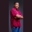Related Tags

c#
communitycreator

# How can to check if two object values are equal in C#Theodore Kelechukwu Onyejiaku

In C#, we can check if two object values are equal with the Equals() method. This method is used to get a Boolean value that indicates whether or not a certain value is equal to another.

### Syntax

public virtual bool Equals (object obj);


### Parameters

obj: This is the object we pass to the Equals() method to check if it is equal to a particular object or not.

### Return value

A boolean value is returned. It returns true if two objects are equal, else, a false is returned.

### Code example

In the example below, we will create some values and check if they are equal to one another, using the Equals() method.

// use System
using System;
// create class
class EqualityChecker
{
// main method
static void Main()
{
// create some values
string name = "Johnny";
string platform = "Edpresso";
bool isGreat = true;
bool isHard = false;
bool isCSharp = true;
int num1 = 3 + 4;   // = 7
int num2 = 5 + 6;   // = 13

// compare them using
// the Equals() method
PrintEquals((string)name, (string)platform);
PrintEquals(name, name);
PrintEquals(isGreat, isHard);
PrintEquals(isGreat, isCSharp);
PrintEquals(num1, num2);
PrintEquals(num1, num1);
}

// check string objects method
static void PrintEquals(object obj1, object obj2){
if(obj1.Equals(obj2)){
Console.WriteLine("Both of the values are equal");
}else{
Console.WriteLine("Both of the values are not equal");
}
}
}
Check if objects are the same in C# using Equals() method

### Explanation

In the code given above:

• In line 10 to line 16: We create strings, boolean values, and integers. They were created so we can check if some of them are equal to each other.

• In line 29, we create a method PrintEquals() that will make use of the Equals() method to compare objects.

• In line 20 to line 25, the method we create is called and the variables we created earlier were passed to the method.

RELATED TAGS

c#
communitycreator

CONTRIBUTORTheodore Kelechukwu Onyejiaku
RELATED COURSES

View all Courses

Keep Exploring

Learn in-demand tech skills in half the time